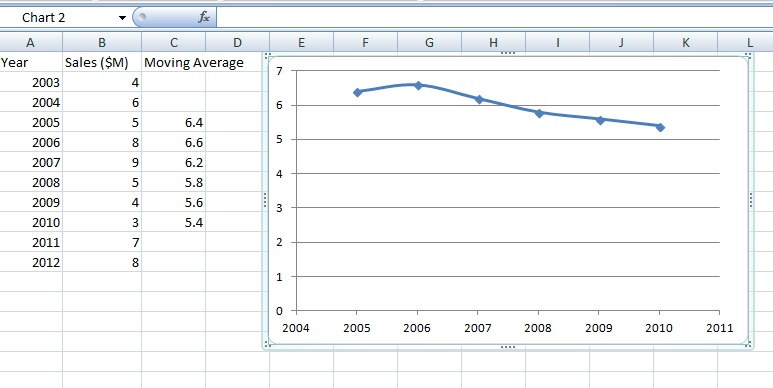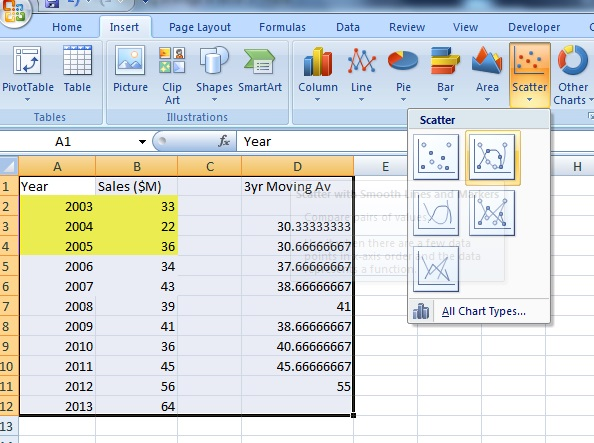# Moving Average: What it is and How to Calculate it

Share on

Contents:

## What is a Moving Average?

Watch the video for an overview and example:

A moving average is a technique to get an overall idea of the trends in a data set; it is an average of any subset of numbers. The moving average is extremely useful for forecasting long-term trends. You can calculate it for any period of time. For example, if you have sales data for a twenty-year period, you can calculate a five-year moving average, a four-year moving average, a three-year moving average and so on. Stock market analysts will often use a 50 or 200 day moving average to help them see trends in the stock market and (hopefully) forecast where the stocks are headed.

An average represents the “middling” value of a set of numbers. The moving average is exactly the same, but the average is calculated several times for several subsets of data. For example, if you want a two-year moving average for a data set from 2000, 2001, 2002 and 2003 you would find averages for the subsets 2000/2001, 2001/2002 and 2002/2003. Moving averages are usually plotted and are best visualized.

### Calculating a 5-Year Moving Average Example

Example Problem: Calculate a five-year moving average from the following data set:

 Year Sales (\$M) 2003 4 2004 6 2005 5 2006 8 2007 9 2008 5 2009 4 2010 3 2011 7 2012 8

The mean (average) sales for the first five years (2003-2007) is calculated by finding the mean from the first five years (i.e. adding the five sales totals and dividing by 5). This gives you the moving average for 2005 (the center year) = 6.4M:

 Year Sales (\$M) 2003 4 2004 6 2005 5 2006 8 2007 9

(4M + 6M + 5M + 8M + 9M) / 5 = 6.4M

The average sales for the second subset of five years (2004 – 2008), centered around 2006, is 6.6M:
(6M + 5M + 8M + 9M + 5M) / 5 = 6.6M

The average sales for the third subset of five years (2005 – 2009), centered around 2007, is 6.6M:
(5M + 8M + 9M + 5M + 4M) / 5 = 6.2M

Continue calculating each five-year average, until you reach the end of the set (2009-2013). This gives you a series of points (averages) that you can use to plot a chart of moving averages. The following Excel table shows you the moving averages calculated for 2003-2012 along with a scatter plot of the data:## Moving Average in Excel 2013: Data Analysis Add-In.

Watch the video or read the steps below:

Excel has a powerful add-in, the Data Analysis Toolpak (how to load the Data Analysis Toolpak) that gives you many extra options, including an automated moving average function. The function not only calculates the moving average for you, it also graphs the original data at the same time, saving you quite a lot of keystrokes.

### Excel 2013: Steps

Step 1: Click the “Data” tab and then click “Data Analysis.”

Step 2: Click “Moving average” and then click “OK.”

Step 3: Click the “Input Range” box and then select your data. If you include column headers, make sure you check the Labels in first Row box.

Step 4: Type an interval into the box. An interval is how many prior points you want Excel to use to calculate the moving average. For example, “5” would use the previous 5 data points to calculate the average for each subsequent point. The lower the interval, the closer your moving average is to your original data set.

Step 5: Click in the “Output Range” box and select an area on the worksheet where you want the result to appear. Or, click the “New worksheet” radio button.

Step 6: Check the “Chart Output” box if you want to see a chart of your data set (if you forget to do this, you can always go back and add it or choose a chart from the “Insert” tab.”

Step 7: Press “OK.” Excel will return the results in the area you specified in Step 6.

## Moving Average in Excel: Using Functions (Non Data Analysis Option)

Watch the video for the steps:

## Steps

Example problem: Calculate the three-year moving average in Excel for the following sales data: 2003(\$33M), 2004(\$22M), 2005(\$36M), 2006(\$34M), 2007(\$43M), 2008(\$39M), 2009(\$41M), 2010(\$36M), 2011(\$45M), 2012(\$56M), 2013(\$64M).

Step 1: Type your data into two columns in Excel. The first column should have the year and the second column the quantitative data (in this example problem, the sales figures). Make sure there are no blank rows in your cell data.

Step 2: Calculate the first three-year average (2003-2005) for the data. For this sample problem, type “=(B2+B3+B4)/3” into cell D3.

Step 3: Drag the square in the bottom right corner down to move the formula to all cells in the column. This calculates averages for successive years (e.g. 2004-2006, 2005-2007).

Step 4: (Optional) Create a graph. Select all of the data in the worksheet. Click the “Insert” tab, then click “Scatter,” then click “Scatter with smooth lines and markers.” A graph of your moving average will appear on the worksheet.That’s it!

Check out our YouTube channel for more stats help and tips!

## References

Salkind, N. (2016). Statistics for People Who (Think They) Hate Statistics: Using Microsoft Excel 4th Edition.

CITE THIS AS:
Stephanie Glen. "Moving Average: What it is and How to Calculate it" From StatisticsHowTo.com: Elementary Statistics for the rest of us! https://www.statisticshowto.com/probability-and-statistics/statistics-definitions/moving-average/
---------------------------------------------------------------------------Need help with a homework or test question? With Chegg Study, you can get step-by-step solutions to your questions from an expert in the field. Your first 30 minutes with a Chegg tutor is free!